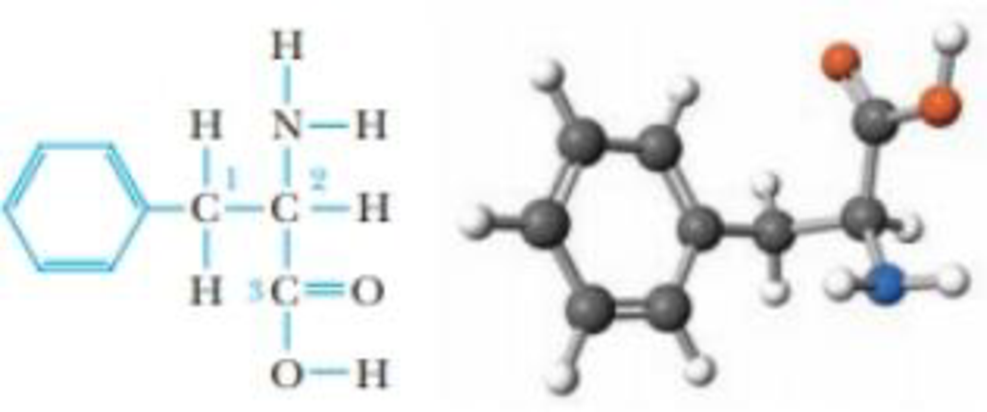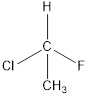Chapter 23, Problem 65PS

Chapter
Section
Textbook Problem

The structure of phenylalanine, one of the 20 amino acids that make up proteins, is drawn below (without lone pairs of electrons). The carbon atoms are numbered for the purpose of this question.(a) What is the geometry of C3?(b) What is the O—C—O bond angle?(c) Is this molecule chiral? If so, which carbon atom is chiral?(d) Which hydrogen atom in this compound is acidic?(a)

Interpretation Introduction

Interpretation:

The geometry of C3 has to be stated.

Concept introduction:

Hybridization is the mixing of valence atomic orbitals to get equivalent hybridized orbitals that having similar characteristics and energy.

Geometry of a molecule can be predicted by knowing its hybridization.

sp3  hybrid orbitals is produced by hybridization of single s-orbital and three p-orbital. It has tetrahedral geometry

sp2  hybrid orbitals is produced by hybridization between one s-orbital and two p-orbitals. It has trigonal-planar geometry

sp  hybrid orbitals is produced by hybridization of single s-orbital and single p-orbital. It has linear geometry

Explanation

The C3 carbon atom is sp2 hybridized and three groups are attached to C3 carbon atom

(b)

Interpretation Introduction

Interpretation:

The bond angle OCO has to be determined.

Concept introduction:

Hybridization is the mixing of valence atomic orbitals to get equivalent hybridized orbitals that having similar characteristics and energy.

Geometry of a molecule can be predicted by knowing its hybridization.

sp3  hybrid orbitals is produced by hybridization of single s-orbital and three p-orbital. It has tetrahedral geometry

sp2  hybrid orbitals is produced by hybridization between one s-orbital and two p-orbitals. It has trigonal-planar geometry

sp  hybrid orbitals is produced by hybridization of single s-orbital and single p-orbital. It has linear geometry

(c)

Interpretation Introduction

Interpretation:

The chirality of this molecule has to be checked and also the chiral carbon atom has to be identified.

Concept introduction:

A chiral carbon is the carbon atom which is substituted to four different groups. Due to presence of chiral carbon a molecule become chiral and it gives optical isomers also that is R and S isomers. For example,All the substituents are different hence is is a chiral carbon and so molecule.

(d)

Interpretation Introduction

Interpretation: The most acidic hydrogen atom in the given compound has to be identified.

Concept introduction:

Any chemical substance can be classified as an acid or base by using Lewis acid base theory. Further, the acidic and basic nature of the substance can be analyzed through the ability of molecules to accept and donate electrons.

According to Lewis acid -base theory,

An acid is a substance that accepts a pair of electrons.

A base is a substance that donates a pair of electrons

Still sussing out bartleby?

Check out a sample textbook solution.

See a sample solution

The Solution to Your Study Problems

Bartleby provides explanations to thousands of textbook problems written by our experts, many with advanced degrees!

Get Started

Vitamin A supplements can help treat acne. T F

Nutrition: Concepts and Controversies - Standalone book (MindTap Course List)

How do eating disorders affect health?

Understanding Nutrition (MindTap Course List)

________ is lifes primary source of energy. a. Food b. Water c. Sunlight d. ATP

Biology: The Unity and Diversity of Life (MindTap Course List)